c++ eigen库
• 这是我在做项目时，给下一届接手的人写的一个关于Eigen库的快速上手手册，主要是针对于项目的应用来写的。当时使用Eigen库的目的是，将Matlab写的，LPCC和MFCC两种声音识别算法，十字形声阵列的MUSIC定位算法，和SVM...Eigen库
• Eigen适用范围广，支持包括固定大小、任意大小的所有矩阵操作，甚至是稀疏矩阵；...Eigen支持多种编译环境，开发人员对中的实例在多种编译环境下经过测试，以保证其在不同编译环境下的可靠性和实用性。
• 主要为大家详细介绍了C++ Eigen库计算矩阵特征值及特征向量，具有一定的参考价值，感兴趣的小伙伴们可以参考一下
• eigen库.zip

2019-07-03 10:41:03
本资源来自于eigen官方网站下载，可以用于矩阵运算，本人在自己的软件工程中，应用成功。C++
• 使用Eigen库实现SVD的完整代码，在ubuntu下的develop已经验证，直接可运行。
• pca算法，也叫主成分分析法，能够对一个多样本的多维特征向量构成的矩阵进行分析，分析主成分并去除维度之间的相关性，使线性相关的向量组变成线性无关的向量组。 并且可以对样本进行降维，降高维向量映射到低维度...
• C ++上的卡尔曼滤波器（本征） 这是使用库在C ++中实现的基本卡尔曼滤波器实现。 它可以直接实现算法，如或在此演示。 该代码的整体结构是从借用，并将其扩展...注意：您可能必须在CMakeLists.txt指定Eigen库的路径。
• Eigen的C++，配置了就可以用，亲测好用。VS2010和VS2015都试过了。Eigen
• 本征 具有Platformio支持的ARM MCU的Eigen库已通过Arduino Due（“ mbed” ststm32 MCU）进行了测试C++
• Eigen是一个高层次的C ++，有效支持线性代数，矩阵和矢量运算，数值分析及其相关的算法。Eigen是一个开源，是ceres solver必备的eigen ceres
•eigen库
• eigen3源码操作示例程序vs2015程序示例,带说明文档，保证通俗易懂，迅速走入eigen开发大门
• C++调用Eigen库技巧的直观理解 包含常用的Eigen库函数及使用方法C++ Eigen
• Eigen库的安装

千次阅读 2021-01-08 19:36:31
Eigen 的安装1. Visual Studio 2017 安装 eigen 1.1 下载 eigen 1.2 配置1.3 运行测试 1. Visual Studio 2017 安装 eigen 1.1 下载 eigen eigen官网下载地址 找到自己需要的版本下载，我下载的是3.3.9...

1. Visual Studio 2017 安装 eigen 库

1.1 下载 eigen 库

eigen官网下载地址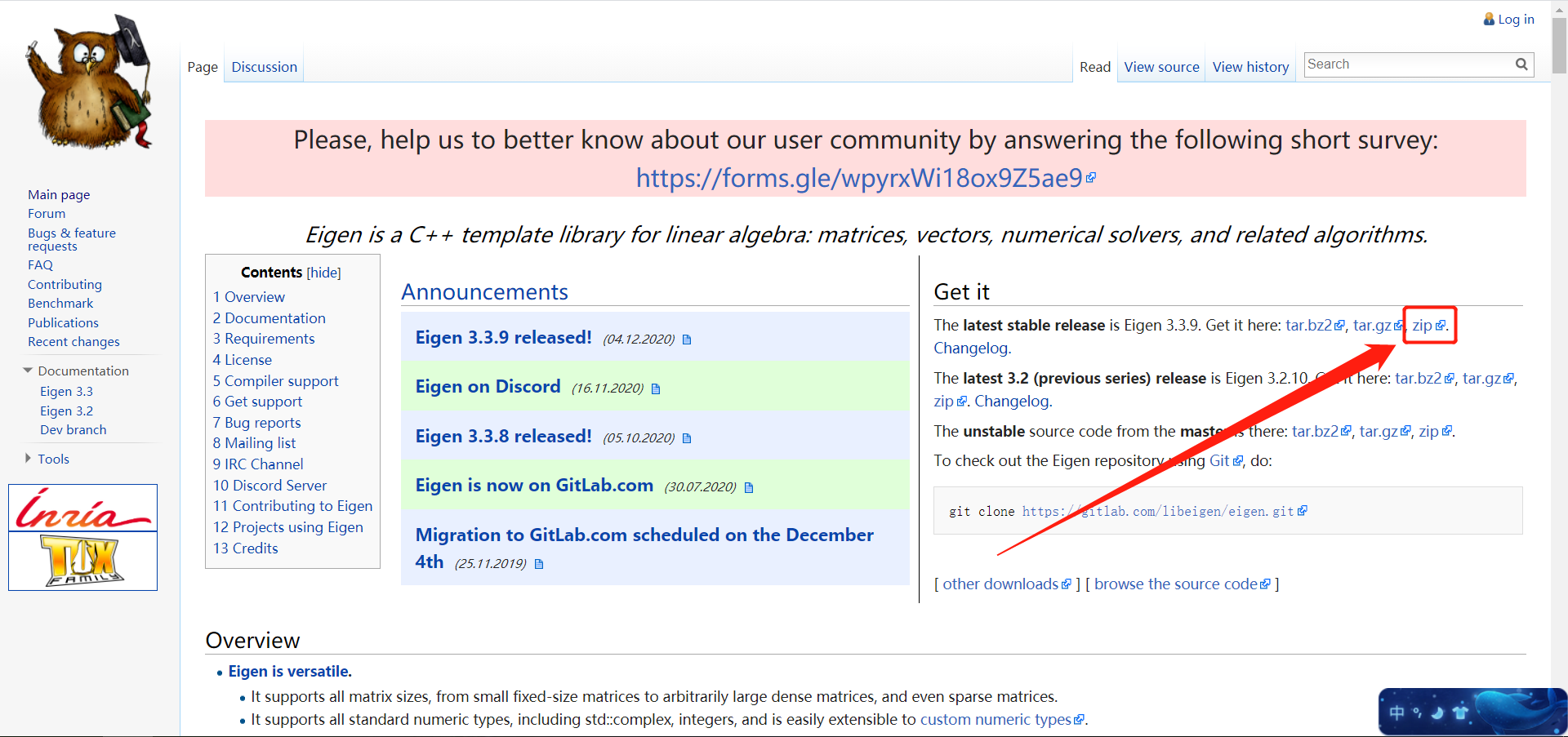找到自己需要的版本下载，我下载的是3.3.9，箭头指向的 zip。
解压缩得到文件eigen-3.3.9，放到自己想放置的路径下（后面会引用此处的路径）。

1.2 配置

在VS 2017中新建一个空项目，取名为“eigen_demo”。输入以下测试代码（官方测试代码）：

#include <iostream>
#include <Eigen/Dense>
using namespace Eigen;
using namespace std;

int main()
{
Matrix2d a;
a << 1, 2,
3, 4;
MatrixXd b(2, 2);
b << 2, 3,
1, 4;
std::cout << "a + b =\n" << a + b << std::endl;
std::cout << "a - b =\n" << a - b << std::endl;
std::cout << "Doing a += b;" << std::endl;
a += b;
std::cout << "Now a =\n" << a << std::endl;
Vector3d v(1, 2, 3);
Vector3d w(1, 0, 0);
std::cout << "-v + w - v =\n" << -v + w - v << std::endl;
#pragma endregion

#pragma  region  Scalar multiplication and division
//	Matrix2d a;  //duplicate definition
a << 1, 2,
3, 4;
//	Vector3d v(1, 2, 3);   //duplicate definition
std::cout << "a * 2.5 =\n" << a * 2.5 << std::endl;
std::cout << "0.1 * v =\n" << 0.1 * v << std::endl;
std::cout << "Doing v *= 2;" << std::endl;
v *= 2;
std::cout << "Now v =\n" << v << std::endl;
#pragma endregion

#pragma  region  Transposition and conjugation
MatrixXcf a_matrix = MatrixXcf::Random(2, 2);
cout << "Here is the matrix a_matrix\n" << a_matrix << endl;
cout << "Here is the matrix a_matrix^T\n" << a_matrix.transpose() << endl;
cout << "Here is the conjugate of a_matrix\n" << a_matrix.conjugate() << endl;
cout << "Here is the matrix a_matrix^*\n" << a_matrix.adjoint() << endl;

//This is the so-called aliasing issue
Matrix2i a_matrix2;
a_matrix2 << 1, 2, 3, 4;
cout << "Here is the matrix a_matrix2:\n" << a_matrix2 << endl;
// a_matrix2 = a_matrix2.transpose(); // !!! do NOT do this !!!
cout << "and the result of the aliasing effect:\n" << a_matrix2 << endl;
#pragma endregion

#pragma  region  Matrix-matrix and matrix-vector multiplication
Matrix2d mat;
mat << 1, 2,
3, 4;
Vector2d u_1(-1, 1), v_1(2, 0);
std::cout << "Here is mat*mat:\n" << mat * mat << std::endl;
std::cout << "Here is mat*u_1:\n" << mat * u_1 << std::endl;
std::cout << "Here is u_1^T*mat:\n" << u_1.transpose()*mat << std::endl;
std::cout << "Here is u_1^T*v:\n" << u_1.transpose()*v_1 << std::endl;
std::cout << "Here is u_1*v_1^T:\n" << u_1 * v_1.transpose() << std::endl;
std::cout << "Let's multiply mat by itself" << std::endl;
mat = mat * mat;
std::cout << "Now mat is mat:\n" << mat << std::endl;
#pragma endregion

#pragma  region  Dot product and cross product
Vector3d v_2(1, 2, 3);
Vector3d w_2(0, 1, 2);
cout << "Dot product: " << v_2.dot(w_2) << endl;
double dp = v_2.adjoint()*w_2; // automatic conversion of the inner product to a scalar
cout << "Dot product via a matrix product: " << dp << endl;
cout << "Cross product:\n" << v_2.cross(w_2) << endl;
#pragma endregion

#pragma region   Basic arithmetic reduction operations
Eigen::Matrix2d mat_3;
mat_3 << 1, 2,
3, 4;
cout << "Here is mat_3.sum():       " << mat_3.sum() << endl;
cout << "Here is mat_3.prod():      " << mat_3.prod() << endl;
cout << "Here is mat_3.mean():      " << mat_3.mean() << endl;
cout << "Here is mat_3.minCoeff():  " << mat_3.minCoeff() << endl;
cout << "Here is mat_3.maxCoeff():  " << mat_3.maxCoeff() << endl;
cout << "Here is mat_3.trace():     " << mat_3.trace() << endl;

Matrix3f m = Matrix3f::Random();
std::ptrdiff_t i, j;
float minOfM = m.minCoeff(&i, &j);
cout << "Here is the matrix m:\n" << m << endl;
cout << "Its minimum coefficient (" << minOfM
<< ") is at position (" << i << "," << j << ")\n\n";
RowVector4i v_4 = RowVector4i::Random();
int maxOfV = v_4.maxCoeff(&i);
cout << "Here is the vector v_4: " << v_4 << endl;
cout << "Its maximum coefficient (" << maxOfV
<< ") is at position " << i << endl;
#pragma endregion

/**************This is the end of example codes in Eigen3 online document. **********************/
system("pause");
}

此时项目默认为“DEBUG”模式，活动平台为“x64"。如果后面更改了模式或平台，均要重新执行全部以下步骤。

鼠标右击项目 -> 选择属性 -> C/C++ -> 常规 -> 附加包含目录，将解压后的库文件夹所在路径:“ \Eigen 库\eigen-3.3.9 ”添加进去，点击确定。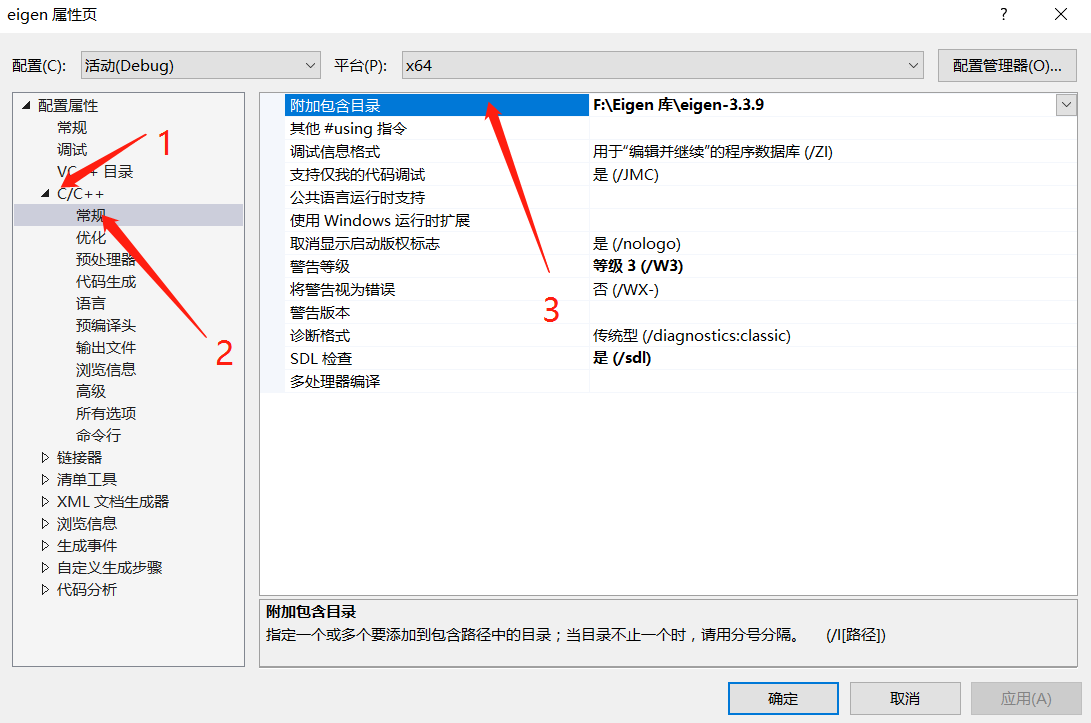再次打开此页面，链接器 -> 常规 -> 附加库目录，将路径“\Eigen 库\eigen-3.3.9”再次添加，点击确定。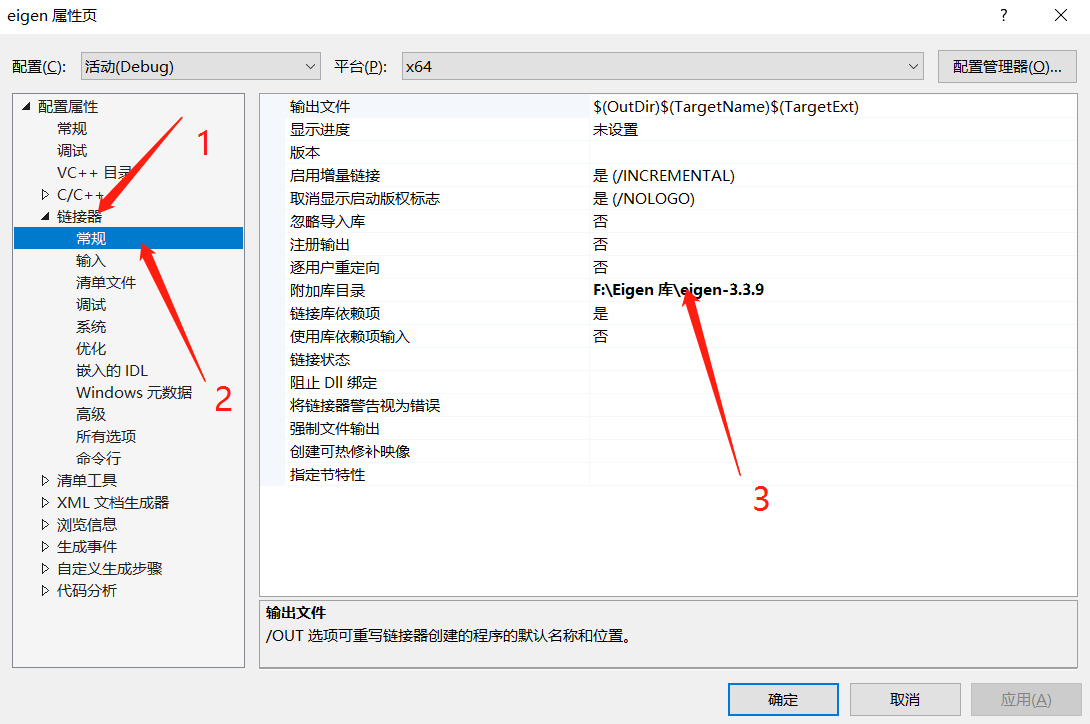1.3 运行测试

运行程序，看到正确运行的输出，Eigen库链接成功！

展开全文• 本篇博文记录了如何使用Eigen库进行基础的矩阵运算，包含了如何创建矩阵、输出矩阵、四则运算、矩阵间运算、矩阵的统计运算、向量的叉乘与点乘的内容。

一、预备工作

1.前言

博主最近在学习高博的《视觉SLAM十四讲》，将对其中一些常用的库文件的使用方式进行记录。本篇博文将简单记录如何使用Eigen库进行基础的矩阵运算。

博主的开发环境为Ubuntu20.04+Clion。

2.安装Eigen3库

一般而言，在Ubuntu安装完成时，将自动安装Eigen3库文件，但是为了防止存在误删或其他原因导致不存在库文件，此处建议大家运行如下指令进行安装库文件

sudo apt-get install -y libeigen3-dev

安装的默认位置一般为：/usr/include/eigen3，可以使用如下指令查看库文件的具体安装位置。

sudo update
locate eigen3

3.新建工程文件

打开Clion，新建工程study_eigen。在该工程下编辑新建的CMakeLists.txt文件，添加如下语句：

include_directories("/usr/include/eigen3")

4.引用头文件

打开main.cpp文件，输入如下内容：

#include<iostream>
using namespace std;

// 导入Eigen库的核心和用于稠密矩阵运算的Dense
#include <Eigen/Core>
#inclued <Eigen/Dense>
using namespace Eigen;

int main(int argc, chr **argv){

return 0;
}

此部分内容引用了Eigen库的核心Core和用于稠密矩阵线性计算的Dense

一、矩阵的定义

1.定义矩阵

在Eigen库中定义矩阵主要采用如下语句：

Matrix<typename Scalar, int RowsAtCompileTime, int ColsAtCompileTime>

其中三个参数分别为数据类型、行数、列数。例如定义一个三行两列的double类型矩阵的语句如下：

Matrix<double, 3, 1> matrix_32d

其中matrix_32d为定义的矩阵的名称，博主习惯用matrix表示该变量为一个矩阵，而32d代表该矩阵为三行两列的double类型矩阵。

2.定义向量

列向量即为N行1列的矩阵，同样行向量为1行N列的矩阵，在Eigen库中的定义方式如下：

// 行向量
Matrix<typename Scalar, int RowsAtCompileTime, 1>
Matrix<typename Scalar, 1, int ColsAtCompileTime>

为了使得向量的定义更加直观，Eigen库使用typedef关键字设置了VectorRowVector用于定义行向量和列向量。

// 定义一个3维float类型列向量
Vector3f vector_31f
// 定义一个2维int类型行向量
RowVector2i vector_12i

同定义矩阵一样，博主习惯将向量定义为vector，其后加上向量的维度和数据类型。

3.常用TypeDef

除了上述使用的定义方式外，Eigen库自定义了大量typedef用于快捷定义矩阵。

// 定义一个3X3的double类型矩阵
Matrix3d matrix_33d
// 定义一个3X3的float类型矩阵
Matrix3f matrix_33f

如果在开发过程中需要定义一个动态的矩阵或则向量可以使用如下方式进行定义

// 定义一个动态大小的矩阵
Matrix<double, Dynamic, Dynamic> matrix_Nd

// 等同于如下定义
MatrixXd matrix_NNd

// 定义一个动态大小的列向量
VectorXi vector_X1i

其中常量Dynamic为Eigen内置的值，为 -1

二、矩阵的属性与输入、输出

1.矩阵的行、列、大小

一个非动态大小的矩阵有自己固有的大小和行列，可以通过如下语句进行调用：

// 矩阵的行数
cout << matrix_33d.rows() << endl;
// 矩阵的列数
cout << matrix_33d.cols() << endl;
// 矩阵的元素个数
cout << matrix_33d.size() << endl;

对于动态大小的矩阵，可以使用resize()对其进行修改个数

// 定义一个初始大小为2*2的动态矩阵
MatrixXd matrix(2, 2);
// 修改其大小为3*3
matrix.resize(3, 3);

2.矩阵的输入

矩阵的输入格式如下：

matrix_33d << 1,2,3,
4,5,6,
7,8,9;

也可以通过索引的方式进行逐个赋值：

// 给第一行第一列的元素赋值
matrix_33d(0,0) = 1

3.矩阵的输出

输出矩阵的元素可以使用迭代的形式进行：

for(int i=0, i<matrix_33d.rows(), i++){
for(int j=0, j<matrix_33d.cols(), j++){
cout << matrix_33d(i, j)<< "\n";
}
cout << endl;
}

此外也可以直接输出整个举矩阵：

cout << matrix_33d <<endl;

4.矩阵初始化

在Eigen中声明矩阵后，可以将其初始化为零一矩阵、单位矩阵等。

# include<iostream>
#include <Eigen/Core>
#include <Eigen/Dense>

using namespace std;
using namespace Eigen;

int main(int argc, char **argv)
{
// 初始化零矩阵
Matrix3d matrix_33d_0 = Matrix3d::Zero();
// 初始化一矩阵
Matrix3d matrix_33d_1 = Matrix3d::Ones();
// 单位矩阵
Matrix3d matrix_33d_I = Matrix3d::Identity();
// 随机矩阵，可填入参数：矩阵行数，矩阵列数
// 生成-1～1的矩阵
Matrix3d matrix_33d_random = Matrix3d::Random();
return 0;
}

三、矩阵的运算

1.基础运算

在Eigen库中，通过对四则算术运算符（+、-、*、/）重载实现了矩阵间的四则运算。由于向量即在某一维度上为1的矩阵，所以除了特殊的运算外不展开说明其他与矩阵相同的运算。

#include <iostream>

using namespace std;

#include <Eigen/Core>
#include <Eigen/Dense>

using namespace Eigen;

int main(int argc, char **argv){
// 定义矩阵
Matrix3d matrix_33d = Matrix3d:Zero();
a << 1, 2,
3, 4;
MatrixXd b(2,2);
b << 2, 3,
1, 4;
// 矩阵间加减
cout << "a + b =\n" << a + b << endl;
cout << "a - b =\n" << a - b << endl;
// 加减复合运算符
cout << "Doing a += b;" << endl;
a += b;
cout << "Now a =\n" << a << endl;
// 取反运算符
cout << -a << endl;
// 矩阵数乘\数除运算
cout << "0.25 * a =\n" << 0.25 * a << endl;
cout << "a / 4 =\n" << a / 4 << endl;
// 矩阵间相乘
cout << "a * a =\n" << a * a << endl;
return 0;
}

需要注意的是Eigen不支持自动进行类型升级，若需要在不同数据类型的矩阵间进行运算，需要进行显式的数据转换。

// float和double类型间的运算需要显式的数据转换
Matrix<double, 2 ,3> result = matrix_23f. cast<double>()  * matrix_33d

2.矩阵自身运算

对于矩阵具备的转置、逆矩阵、共轭矩阵、迹等通过调用对应API即可。

cout << matrix_33d.transpose() << endl;			// 矩阵求转置
cout << matrix_33d.inverse() << endl;			// 矩阵求逆
cout << matrix_33d.conjugate()  << endl;		// 矩阵求共轭
cout << mtrix_33d.adjoint() << endl;			// 矩阵求伴随矩阵
cout << matrix_33d.trace() << endl;				// 矩阵求迹
cout << matrix_33d.determinant() << endl;		// 矩阵行列式

3.向量的叉乘和点乘

在Eigen库中使用dot()cross() 方法来实现向量的叉乘点乘

#include <iostream>
#include <Eigen/Dense>

using namespace Eigen;
using namespace std;

int main(){
Vector3d v(1,2,3);
Vector3d w(0,1,2);

cout << "向量点乘 " << v.dot(w) << endl;
// 等同于将其中一个向量转换为其伴随矩阵，然后两个矩阵相乘
cout << dp << endl;
cout << "向量叉乘\n" << v.cross(w) << endl;

4.矩阵的统计运算

Eigen库自带了用于统计运算的函数，如求和、求最大值、最小值等。

#include <iostream>
#include <Eigen/Dense>

using namespace std;
using namespace Eigen;

int main(){
Matrix2d mat;
mat << 1, 2,
3, 4;
cout << "矩阵元素求和：" << mat.sum() << endl;
cout << "矩阵元素求积：" << mat.prod()      << endl;
cout << "矩阵元素取中位数：" << mat.mean()      << endl;
cout << "矩阵元素取最小值：" << mat.minCoeff()  << endl;
cout << "矩阵元素取最大值：" << mat.maxCoeff()  << endl;
}

5.矩阵的特征值和特征向量

在矩阵运算中，计算矩阵的特征值和特征向量十分重要，Eigen库中提供了如下方法，计算矩阵的特征值。

cout << eigensolver.eigenvalues()	<< endl;       // 求矩阵特征值
cout << eigensolver.eigenvectors() << endl;        // 求矩阵特征向量

四、广播机制

广播构造了一个表达式，其中向量（列或行）通过在一个方向上复制从而形成矩阵。实现广播需要使用方法**.colwise()** 和 .rowwise()

#include <iostream>
#include <Eigen/Dense>

using namespace std;
using namespace Eigen;

int main()
{
MatrixXf mat(2,4);
VectorXf v(2);

mat << 1, 2, 6, 9,
3, 1, 7, 2;

v_1 << 0,
1;

v_2 << 0,1,2,3;

// 列向量广播
mat.colwise() += v_1;

cout << "Broadcasting result: " << endl;
cout << mat << endl;
/*
* 输出结果如下：
* 1 2 6 9
* 4 2 8 3
*/

// 行向量广播
mat.rowwise() += v_2

cout << "Broadcasting result: " << endl;
cout << mat << endl;
/*
* 输出结果如下：
* 1 3  8 12
* 4 3 10  6
*/
}

上述代码即为实现将列向量进行广播，实现与矩阵相加。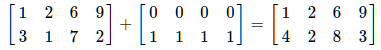展开全文c++
• 环境 VS2017 配置 下载Eigen库 ... 包含目录,添加Eigen库路径 Eigen基本类型使用 #include <iostream> #include <Eigen/Core> //包含Matrix和Array类，基础的线性

VS2017

配置

1. 下载Eigen库 http://eigen.tuxfamily.org/index.php?title=Main_Page
解压至目标路径下
2. 新建项目，文件
3. Debug|x64下添加*.sheet*文件
4. 在属性->配置属性-> vc++目录-> 包含目录,添加Eigen库路径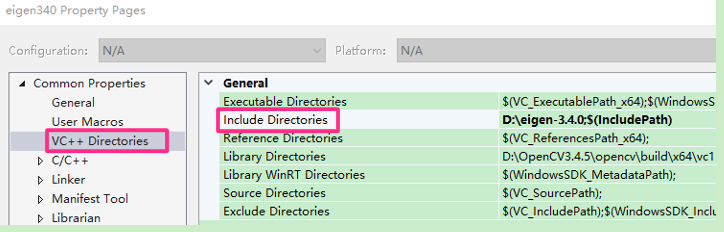Eigen基本类型使用

#include <iostream>
#include <Eigen/Core>	//包含Matrix和Array类，基础的线性代数运算和数组操作
#include<Eigen/Dense>	//包含了Core/Geometry/LU/Cholesky/SVD/QR/Eigenvalues模块
#include <ctime>

using namespace Eigen;
using namespace std;

#define MATRIX_SIZE 50

int main() {
Matrix<float, 2, 3>matrix_23;	// 声明一个2*3的float矩阵
Vector3d v_3d;	//Vector3d 实质上是 Eigen::Matrix<double, 3, 1>，即三维向量
Matrix<float, 3, 1>vd_3d;
Matrix3d matrix_33 = Matrix3d::Zero();	// Matrix3d 实质上是 Eigen::Matrix<double, 3, 3>
Matrix<double, Dynamic, Dynamic>matrix_dynamic;	// 如果不确定矩阵大小，可以使用动态大小的矩阵
MatrixXd matrix_x;

// 输入数据（初始化）
matrix_23 << 1, 2, 3, 4, 5, 6;
cout << "2x3阶矩阵：\n" << matrix_23 << endl << endl;

//用()访问矩阵中的元素
cout << "2x3矩阵：" << endl;
for (int i = 0; i < 2; i++) {
for (int j = 0; j < 3; j++) {
cout << matrix_23(i, j) << " ";
}
cout << endl;
}
cout << endl;

// 矩阵和向量相乘（实际上仍是矩阵和矩阵）
v_3d << 3, 2, 1;
vd_3d << 4, 5, 6;
//显式转换
Matrix<double, 2, 1>result = matrix_23.cast<double>()*v_3d;
cout << "[1,2,3;4,5,6]*[3,2,1]=" << result.transpose() << endl;

Matrix<float, 2, 1> result2 = matrix_23 * vd_3d;
cout << "[1,2,3;4,5,6]*[4,5,6]: " << result2.transpose() << endl << endl;

//打开下面的//后报错 EC2338 YOU_MIXED_MATRICES_OF_DIFFERENT_SIZES
//Eigen::Matrix<double, 2, 3> result_wrong_dimension = matrix_23.cast<double>() * v_3d;

matrix_33 = Matrix3d::Random();      // 随机数矩阵
cout << "random matrix: \n" << matrix_33 << endl << endl;
cout << "转置: \n" << matrix_33.transpose() << endl << endl;  // 转置
cout << "各元素和: " << matrix_33.sum() << endl;      // 各元素和
cout << "trace: " << matrix_33.trace() << endl << endl;      // 迹
cout << "times 10: \n" << 10 * matrix_33 << endl << endl;;    // 数乘
cout << "inverse: \n" << matrix_33.inverse() << endl << endl;  // 逆
cout << "det: " << matrix_33.determinant() << endl;    // 行列式

// 特征值
// 实对称矩阵可以保证对角化成功
cout << "Eigen values = \n" << eigen_solver.eigenvalues() << endl << endl;
cout << "Eigen vectors = \n" << eigen_solver.eigenvectors() << endl << endl;

// 解方程
// 我们求解 matrix_NN * x = v_Nd 这个方程
// N的大小在前边的宏里定义，它由随机数生成
// 直接求逆自然是最直接的，但是求逆运算量大

Matrix<double, MATRIX_SIZE, MATRIX_SIZE>matrix_NN = MatrixXd::Random(MATRIX_SIZE, MATRIX_SIZE);
matrix_NN = matrix_NN * matrix_NN.transpose();	// 保证半正定
Matrix<double, MATRIX_SIZE, 1>v_Nd = MatrixXd::Random(MATRIX_SIZE, 1);

clock_t time_stt = clock();  // 计时
// 直接求逆
Matrix<double, MATRIX_SIZE, 1> x = matrix_NN.inverse() * v_Nd;
cout << "time of normal inverse is "
<< 1000 * (clock() - time_stt) / (double)CLOCKS_PER_SEC << "ms" << endl;
cout << "x = " << x.transpose() << endl << endl;

// 通常用矩阵分解来求，例如QR分解，速度会快很多
time_stt = clock();
x = matrix_NN.colPivHouseholderQr().solve(v_Nd);
cout << "time of Qr decomposition is "
<< 1000 * (clock() - time_stt) / (double)CLOCKS_PER_SEC << "ms" << endl;
cout << "x = " << x.transpose() << endl << endl;

// 对于正定矩阵，还可以用cholesky分解来解方程
time_stt = clock();
x = matrix_NN.ldlt().solve(v_Nd);
cout << "time of ldlt decomposition is "
<< 1000 * (clock() - time_stt) / (double)CLOCKS_PER_SEC << "ms" << endl;
cout << "x = " << x.transpose() << endl << endl;

system("pause");
return 0;
}

展开全文线性代数 机器学习
• eigen库 Eigen库的使用 有关eigen库的一些基本使用方法 Eigen库 矩阵基本操作：转置矩阵，逆矩阵，伴随矩阵，特征值 Eigen介绍及简单使用 Eigen（3）矩阵Matrix及其简单操作

Eigen是可以用来进行线性代数、矩阵、向量操作等运算的C++库，它里面包含了很多算法。在用C++写运动控制算法（例如MPC控制算法）的时候,由于算法包括矩阵运算，c++对矩阵的运算支持不是很好，而Eigen（读作 ['aɪgən]）是一个简单易用的用于矩阵运算的库。可以利用Eigen库进行运算，并且可以直接求解矩阵的逆、转置、伴随矩阵等，不需要利用数组进行计算了，比较方便快捷。

一.eigen库的安装与VS配置

1. Eigen库的安装
首先在官网下载Eigen库，库的官网下载地址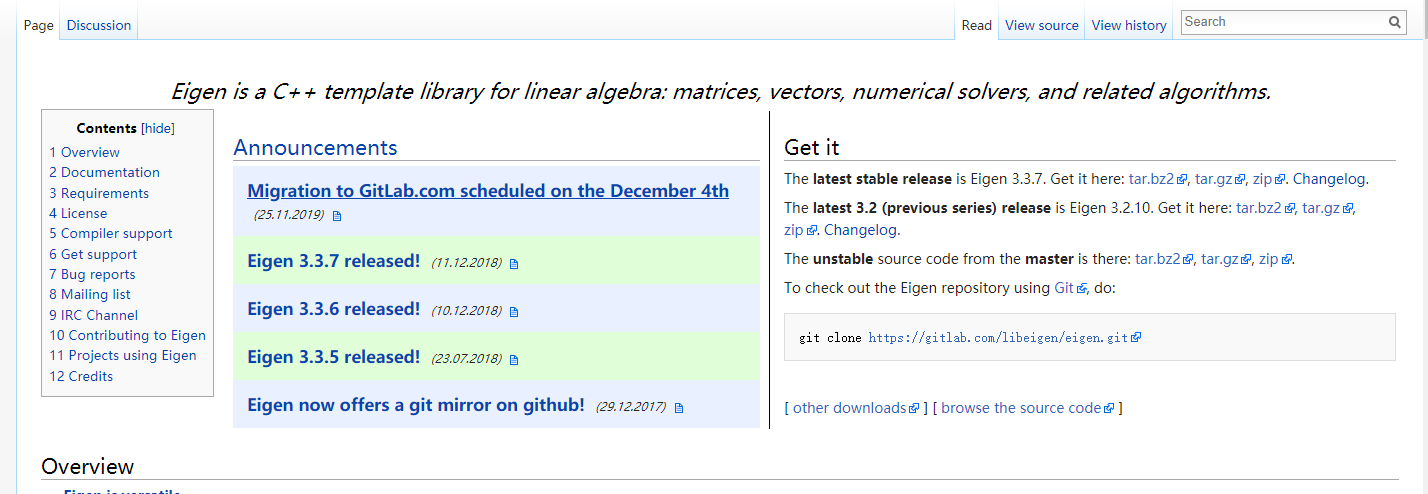解压缩文件可以得到以下的文件2. VS 的附加依赖库的配置
由于Eigen都是由头文件组成的，所以包含相应的库路径即可。
在VS2017运行环境下，首先包含相应的库路径
**在项目上右键，找到属性，添加相应的库路径即可，注意我这里包含到路径到Eigen这个文件夹，所以在包含头文件时，#include <Dense即可，不需要写#include<Eigen/Dense>，如果没有的话需要包含到Eigen/Dense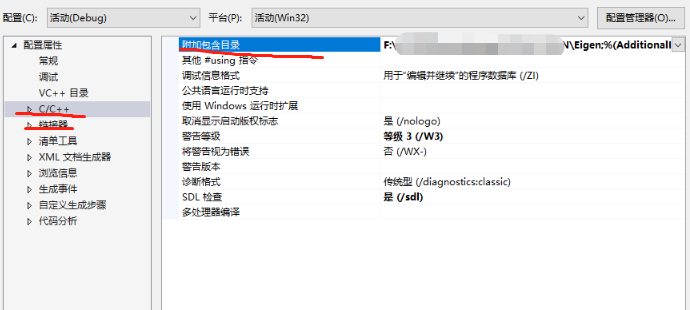如果是在ROS系统里面，在你的项目的CMakeLists中包含相应的库路径即可。

二.eigen库的简单使用

矩阵的定义：Eigen中关于矩阵类的模板函数中，共有六个模板参数，常用的只有前三个。其前三个参数分别表示矩阵元素的类型、行数和列数。
矩阵定义时可以使用Dynamic来表示矩阵的行列数为未知。
1.程序的声明
在添加了相应的路径之后，在程序开头写这几行即可使用了

#include <iostream>
#include <Dense>   //或者是 #include "Eigen/Dense"
using Eigen::MatrixXd;
using namespace std;

声明一个矩阵：
矩阵类型：Eigen中的矩阵类型一般都是用类似MatrixXXX来表示，可以根据该名字来判断其数据类型，比如”d”表示double类型，”f”表示float类型，”i”表示整数，”c”表示复数；Matrix2f，表示的是一个2*2维的，其每个元素都是float类型。

MatrixXd P(3, 6);  //说明矩阵成员是double  类型的
MatrixXf s(3, 1);  //说明矩阵成员是float   类型的
MatrixXd v(6, 1);

第一行表示生成一个3行6列的矩阵

2.矩阵、向量的初始化
向矩阵输入数据,顺序输入即可（逗号初始化
动态矩阵和静态矩阵：动态矩阵是指其大小在运行时确定，静态矩阵是指其大小在编译时确定。
MatrixXd：表示任意大小的元素类型为double的矩阵变量，其大小只有在运行时被赋值之后才能知道。
Matrix3d：表示元素类型为double大小为3*3的矩阵变量，其大小在编译时就知道。
在Eigen中行优先的矩阵会在其名字中包含有row，否则就是列优先。Eigen中的向量只是一个特殊的矩阵，其维度为1而已。

Matrix3f m;
m << 1, 2, 3,
4, 5, 6,
7, 8, 9;
std::cout << m;

输入常见矩阵
矩阵元素的访问：在矩阵的访问中，行索引总是作为第一个参数，Eigen中矩阵、数组、向量的下标都是从0开始。矩阵元素的访问可以通过”()”操作符完成。例如m(2, 3)既是获取矩阵m的第2行第3列元素。获取元素：通过中括号获取元素，对于矩阵是：（行，列）；对于向量，只是传递它的索引，以0为起始。

#include<iostream>
#include<Eigen/Dense>
//matrix
int main1()
{
Eigen::MatrixXd m(2, 2);
m(0, 0) = 1;
m(0, 1) = 2;
m(1, 0) = 3;
m(1, 1) = 4;
std::cout << "m= " << std::endl << m << std::endl;
return 0;
}

设置矩阵的元素：在Eigen中重载了”<<”操作符，通过该操作符即可以一个一个元素的进行赋值，也可以一块一块的赋值。另外也可以使用下标进行赋值。
重置矩阵大小：当前矩阵的行数、列数、大小可以通过rows()、cols()和size()来获取，对于动态矩阵可以通过resize()函数来动态修改矩阵的大小。注意：(1)、固定大小的矩阵是不能使用resize()来修改矩阵的大小；(2)、resize()函数会析构掉原来的数据，因此调用resize()函数之后将不能保证元素的值不改变；(3)、使用”=”操作符操作动态矩阵时，如果左右两边的矩阵大小不等，则左边的动态矩阵的大小会被修改为右边的大小。
如何选择动态矩阵和静态矩阵：对于小矩阵(一般大小小于16)使用固定大小的静态矩阵，它可以带来比较高的效率；对于大矩阵(一般大小大于32)建议使用动态矩阵。注意：如果特别大的矩阵使用了固定大小的静态矩阵则可能会造成栈溢出的问题。

int main
{
MatrixXf m1(3,4);   //动态矩阵，建立3行4列。
MatrixXf m2(3,3);
Vector3f v1;		//若是静态数组，则不用指定行或者列
/* 初始化 */
m1 = MatrixXf::Zero(3,4);		//用0矩阵初始化,要指定行列数
m2 = MatrixXf::Identity(3,3);	//用单位矩阵初始化
v1 = Vector3f::Zero();			//同理，若是静态的，不用指定行列数
m1 << 1,0,0,1,      //也可以以这种方式初始化
1,5,0,1,
0,0,9,1;
//向量初始化，与矩阵类似
Vector3d v3(1,2,3);
VectorXf vx(30);
}

列向量

typedef Matrix<float, 3, 1> Vector3f;

行向量

typedef Matrix<int, 1, 2> RowVector2i;

3.矩阵基础操作（加减乘除）
eigen重载了基础的+ - * / += -= = /= 可以表示标量和矩阵或者矩阵和矩阵

#include <iostream>
#include <Eigen/Dense>
using namespace Eigen;
int main()
{
//单个取值，单个赋值
double value00 = staMat(0,0);
double value10 = staMat(1,0);
staMat(0,0) = 100;
std::cout << value00 <<value10<<std::endl;
std::cout <<staMat<<std::endl<<std::endl;
//加减乘除示例 Matrix2d 等同于 Matrix<double,2,2>
Matrix2d a;
a << 1, 2,
3, 4;
MatrixXd b(2,2);
b << 2, 3,
1, 4;

Matrix2d c = a + b; //矩阵的加法
std::cout<< c<<std::endl;
c = a - b;  //矩阵的减法
std::cout<<c<<std::endl;
c = a * 2;//矩阵的乘法
std::cout<<c<<std::endl;
c = 2.5 * a;//矩阵的乘法
std::cout<<c<<std::endl;
c = a / 2;//矩阵的除法
std::cout<<c<<std::endl;
c = a * b;//矩阵的乘法
std::cout<<c<<std::endl;

4.C++数组和矩阵的转换
使用Map函数，可以实现Eigen的矩阵和c++中的数组直接转换,如下：

int i;
//数组转矩阵
double *aMat = new double;
for(i =0;i<20;i++)
{
aMat[i] = rand()%11;
}
//静态矩阵，编译时确定维数 Matrix<double,4,5>
Eigen:Map<Matrix<double,4,5> > staMat(aMat);

//输出
for (int i = 0; i < staMat.size(); i++)
std::cout << *(staMat.data() + i) << " ";
std::cout << std::endl << std::endl;

//动态矩阵，运行时确定 MatrixXd
Map<MatrixXd> dymMat(aMat,4,5);

//输出，应该和上面一致
for (int i = 0; i < dymMat.size(); i++)
std::cout << *(dymMat.data() + i) << " ";
std::cout << std::endl << std::endl;

//Matrix中的数据存在一维数组中，默认是行优先的格式，即一行行的存
//data()返回Matrix中的指针
dymMat.data();

5.重置矩阵的大小，重新设置矩阵尺寸
重置矩阵大小：当前矩阵的行数、列数、大小可以通过rows()、cols()和size()来获取，对于动态矩阵可以通过resize()函数来动态修改矩阵的大小。注意：(1)、固定大小的矩阵是不能使用resize()来修改矩阵的大小；(2)、resize()函数会析构掉原来的数据，因此调用resize()函数之后将不能保证元素的值不改变；(3)、使用”=”操作符操作动态矩阵时，如果左右两边的矩阵大小不等，则左边的动态矩阵的大小会被修改为右边的大小。
matrix的大小可以通过rows()、cols()、size()获取，resize()可以重新调整动态matrix的大小

#include <iostream>
#include <Eigen/Dense>
using namespace Eigen;
int main()
{
MatrixXd m(2,5);
m.resize(4,3);
std::cout << "The matrix m is of size "
<< m.rows() << "x" << m.cols() << std::endl;
std::cout << "It has " << m.size() << " coefficients" << std::endl;
VectorXd v(2);
v.resize(5);
std::cout << "The vector v is of size " << v.size() << std::endl;
std::cout << "As a matrix, v is of size "
<< v.rows() << "x" << v.cols() << std::endl;
}

对应的输出为：

The matrix m is of size 4x3
It has 12 coefficients
The vector v is of size 5
As a matrix, v is of size 5x1

6.矩阵的常用矩阵：转置、伴随、行列式、特征值等
小矩阵（4 * 4及以下）eigen会自动优化，默认采用LU分解，效率不高
转置、伴随、行列式、逆矩阵

#include <iostream>
#include <Eigen/Dense>
using namespace std;
using namespace Eigen;
int main()
{
Matrix2d c;
c << 1, 2,
3, 4;
//转置、伴随
std::cout<<c<<std::endl<<std::endl;
std::cout<<"转置\n"<<c.transpose()<<std::endl<<std::endl;
//逆矩阵、行列式
std::cout << "行列式： " << c.determinant() << std::endl;
std::cout << "逆矩阵\n" << c.inverse() << std::endl;
}

计算特征值和特征向量

#include <iostream>
#include <Eigen/Dense>
using namespace std;
using namespace Eigen;
int main()
{
//特征向量、特征值
std::cout << "Here is the matrix A:\n" << a << std::endl;
if (eigensolver.info() != Success) abort();
std::cout << "特征值:\n" << eigensolver.eigenvalues() << std::endl;
std::cout << "Here's a matrix whose columns are eigenvectors of A \n"
<< "corresponding to these eigenvalues:\n"
<< eigensolver.eigenvectors() << std::endl;
}

感谢下面博主的分享：
Eigen介绍及简单使用（基本的知识的了解）
有关eigen库的一些基本使用方法（基本的操作实例）
Eigen（3）矩阵Matrix及其简单操作

展开全文• 使用vs2017和cmake编译的Eigen3.3.9的，32位64位通用
• 1. 下载并按照Eigen库 首先打开Eigen官网（https://eigen.tuxfamily.org/index.php）。找到合适的Eigen版本，右键点击tar.gz压缩包，点击复制链接。如下例图 之后在CentOS终端粘贴，下载Eigen文件： wget ...centos eigen 安装 错误
• Eigen库介绍及使用入门

千次阅读 2019-12-16 10:56:42
为了将Matlab写的运动学程序转化为C++所编写的dll，需要用用到矩阵库Eigen，Eigen库是一个使用C++源码编写的矩阵库，基本上能满足计算中所需要用到的运算，下面介绍一些库的入门学习。 1.首先是关于固定大小矩阵，...
• VS2017 Eigen库环境配置 添加Eigen库

千次阅读 多人点赞 2020-07-17 17:43:36
C++问题五 Eigen库环境配置 环境：Windows10 VS2017 在C++下，有时候我们需要实现一些矩阵的算法，这个时候我们需要去添加一些库，实现Matlab中矩阵运算的库函数有很多，在这里我添加的库位Eigen。 第一步： 下载...c++
• 参考 Eigen官网教程 ... 简介 Eigen是C++中可以用来调用并进行矩阵计算的一个库，简单了说它就是一个c++版本的matlab包。 安装 Eigen是一个纯用头文件...要使用Eigen库，需要在cmake工程中的CMakeLists.txt文件中指定Eigubuntu matlab linux
• Eigen库中常用函数： 单位矩阵的函数为eye(row,col) 对角线为1的任意大小的矩阵Identity(5, 4) 矩阵的求逆：matrix.inverse() 生成随机的矩阵 matrix33 = Eigen::Matrix3d::Random(); 转置 matrix33.transpose() ...矩阵
• 在slam中最重要的就是Eigen库，在高博的书上使用的是KDdevelop，我对vscode情有独钟想要在上面学习——死磕就完事了。如果遇到vscode对eigen库报错这个问题请直接拉到下面看。 一、现在终端mkdir （文件夹名字），...vscode
• Ubuntu安装Eigen库一、Eigen库的下载二、Eigen库的安装1.解压缩2.编译3.安装三、复制Eigen库到 /usr/local/include 中安装成功！！！ 一、Eigen库的下载 点击此处跳转下载CSDN中上传的资源 或者 点击此处跳转百度...ubuntu
• 前言：Eigen 是一个线性算术的C++模板，包括：vectors, matrices, 开源以及相关算法。功能强大、快速、优雅以及支持多平台，可以使用该来方便处理一些矩阵的操作，达到类似matlab那样的快捷。现在已经发展到...
• ubuntu18.04安装eigen库 eigen相对来说很容易装，但是不同版本在使用时会对程序的效果有很大影响。因此在安装之前一定要选对版本。 源码编译 从github上下载eigen库的包： git clone ...自动驾驶 c++ 机器学习...